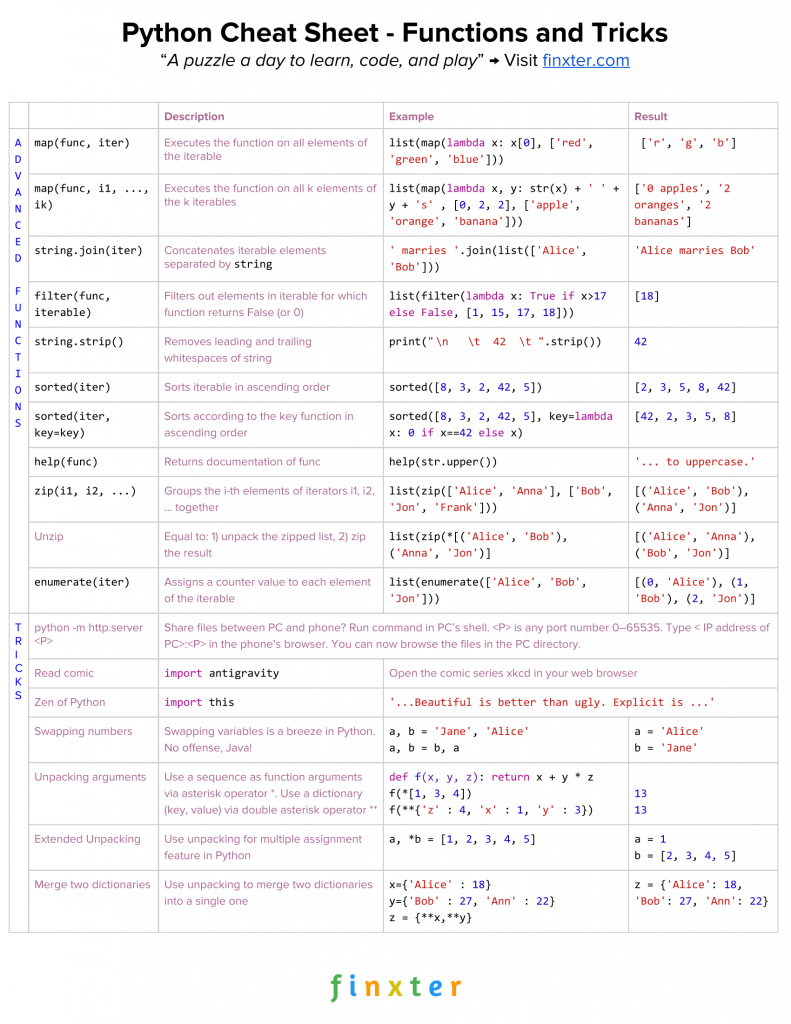# Python Functions and Tricks Cheat Sheet

5/5 - (1 vote)

Python cheat sheets are the 80/20 principle applied to coding: learn 80% of the language features in 20% of the time.
Download and pin this cheat sheet to your wall until you feel confident using all these tricks.Try It Yourself:

Exercise: Modify each function and play with the output!

Here’s the code for copy&paste:

```# 1. map(func, iter)
m = map(lambda x: x, ['red', 'green', 'blue'])
print(list(m))
# ['r', 'g', 'b']

# 2. map(func, i1, ...,ik)
l1 = [0, 2, 2]
l2 = ['apple', 'orange', 'banana']
m = map(lambda x, y: str(x) + ' ' + str(y) + 's', l1, l2)
print(list(m))
# ['0 apples', '2 oranges', '2 bananas']

# 3. string.join(iter)
print(' marries '.join(['Alice', 'Bob']))
# Alice marries Bob

# 4. filter(func, iterable)
print(list(filter(lambda x: x>17, [1, 15, 17, 18])))
# 

# 5. string.strip()
print("    \n   \t  42  \t ".strip())
# 42

# 6. sorted(iter)
print(sorted([8, 3, 2, 42, 5]))
# [2, 3, 5, 8, 42]

# 7. sorted(iter, key=key)
print(sorted([8, 3, 2, 42, 5], key=lambda x: 0 if x==42 else x))
# [42, 2, 3, 5, 8]

# 8. help(func)
help(str.upper)
'''
Help on method_descriptor:

upper(self, /)
Return a copy of the string converted to uppercase.
'''

# 9. zip(i1, i2, ...)
print(list(zip(['Alice', 'Anna'], ['Bob', 'Jon', 'Frank'])))
# [('Alice', 'Bob'), ('Anna', 'Jon')]

# 10. Unzip
print(list(zip(*[('Alice', 'Bob'), ('Anna', 'Jon')])))
# [('Alice', 'Anna'), ('Bob', 'Jon')]

# 11. Enumerate
print(list(enumerate(['Alice', 'Bob', 'Jon'])))
# [(0, 'Alice'), (1, 'Bob'), (2, 'Jon')]
```

The following tutorials address those functions in great detail (including videos on the most important functions).

Related Articles: